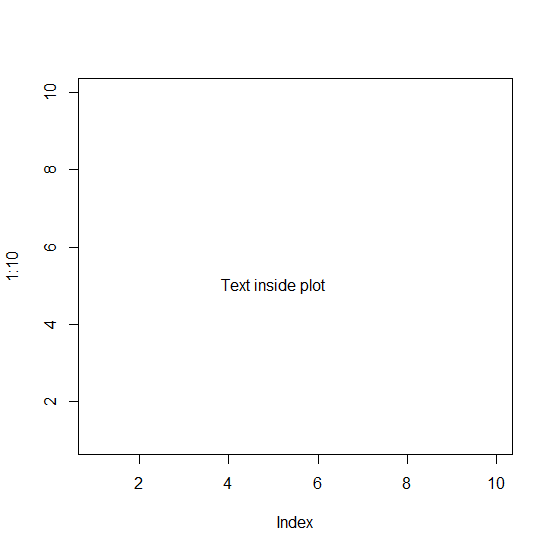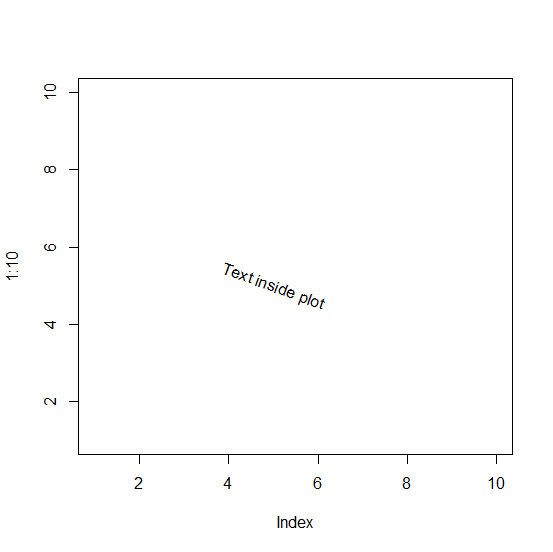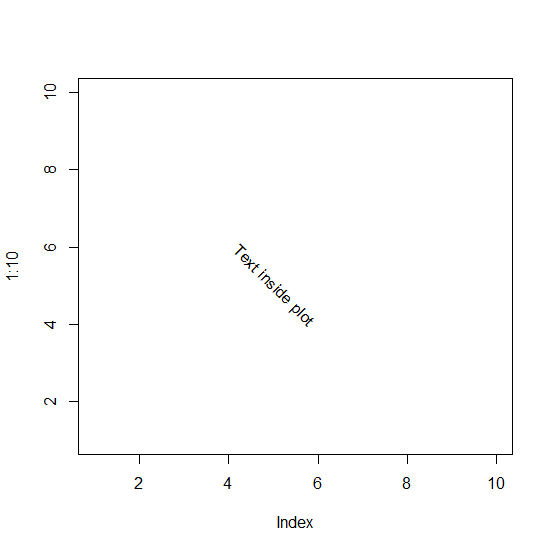# How to rotate text in base R plot?

To write the text in a base R plot, firstly we should create a blank chart by using type="n" if we want to plot only text then the text should be introduced with the help of text function. Now, if we want to rotate the text value then srt argument can be used. For example, if we want to rotate the text to 45 degree then srt = -45 can be used.

## Example1

Live Demo

> plot(1:10,type="n")
> text(x=5,y=5,"Text inside plot")

## Output## Example2

Live Demo

> plot(1:10,type="n")
> text(x=5,y=5,"Text inside plot",srt=-20)

## Output## Example3

Live Demo

> plot(1:10,type="n")
> text(x=5,y=5,"Text inside plot",srt=-45)

## Output